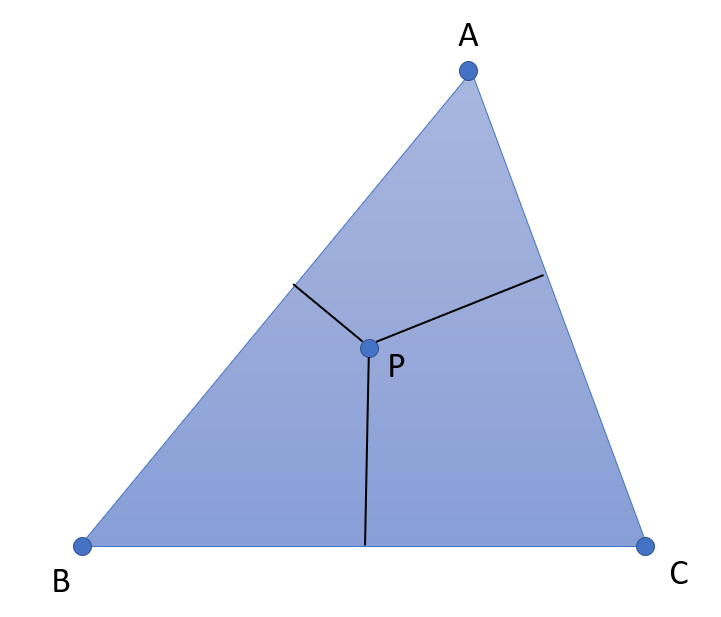# Trilinear Coordinates

Trilinear coordinates, introduced by Möbius in 1827, is a way to identify a point in a plane with respect to a triangle. The coordinates are defined by measuring signed distances from a triangle’s sides.Basically, it is a coordinate system where a triangle assigns three coordinates to a point P :

• α = [P, BC]
• β = [P, AC]
• γ = [P, AB].

The three numbers are an ordered triple (α, β, γ), where each is proportional to the directed distance from P to one of the triangle’s side lines .

## Example of Use

Trilinear coordinates are used to study the geometric properties of triangles using algebra. In real life, let’s say you are studying a mixture of three components a, b, and c. Point P could represent a mixture with percent composition represented by the trilinear coordinates (α, β, γ).

Let’s say a triangle ABC has a perpendicular from vertex A to base BC, as shown in the above image. If the perpendicular A = 100 units (representing 100 percent of the mixture), then a point P with trilinear coordinates (α, β, γ) will satisfy α + β + γ = 100.

## Trilinear Coordinates vs. Barycentric Coordinates

Other names for trilinear coordinates include trilinears, homogeneous trilinear coordinates, and barycentric coordinates (a barycenter is another name for center of mass). Although the names are often used synonymously, there is a subtle difference between barycentric coordinates and trilinears :

• The barycentric coordinates of point P are an ordered triple where P is the centroid consisting of three masses at vertices A, B, and C.
• Trilinear coordinates of point P are an ordered triple that is proportional to the directed distance from P to the sides.

## The Center Function and Trilinear Coordinates

A center function (also called a triangle center function, symmetric triangle center function or simply a center) gives the trilinear coordinates of a triangle’s center. The function’s three variables {a, b, c} or {α, β γ} correspond to angles or sides.

## What is a Triangle Center?

A triangle center is a point defined in terms of a triangle’s side lengths and angles and for which a center function exists. A major triangle center is one where the function α = f(A, B, C) is only a function of angle A.

It sounds simple, but don’t be fooled into thinking there’s just one center to a triangle. A “center” can be defined in many ways. For example, the centroid is the intersection of the triangle medians, and the circumcenter is the center of the circle inscribing the triangle.In fact, there are thousands of different triangle centers including the Fermat point, which has the center function α = csc(A + ⅓π) and the Far-Out point, with function α = a(b4 + c4 – a4 – b2c2).

## Properties of the Triangle Center Function

The triangle center function is a homogeneous function (i.e. with variables that increase by the same proportion). In notation, that’s:
f ( t a, t b, t c) = tn f (a, b, c).
Where n is a constant.

The function is also nonzero, meaning that it can’t have values equal to zero. This makes sense, because a non-zero value of a triangle would result in a non-triangle shape: a line, a point, or no shape at all.

The triangle center function has bisymmetry (two planes of symmetry at right angles) in the second and third variables. In notation:
f(a, b, c) = f(a, c, b).

## References

 22 Trilinear Coordinates. Retrieved May 15, 2022 from: http://www.mcs.uvawise.edu/msh3e/resources/geometryBook/22TrilinearCoordinates.pdf
 Yiu, P. The uses of homogeneous barycentric coordinates in plane Euclidean geometry.
 Boju, V. & Funar, L. (2007). The Math Problems Notebook. Birkhäuser Boston

CITE THIS AS:
Stephanie Glen. "Trilinear Coordinates" From StatisticsHowTo.com: Elementary Statistics for the rest of us! https://www.statisticshowto.com/trilinear-coordinates/